# tidydice

### Introduction

A basic understanding of probability and statistics is crucial for data understanding and discovery of meaningful patterns. A great way to teach probability and statistics is to start with an experiment, like rolling a dice or flipping a coin.

This package simulates rolling a dice and flipping a coin. Each experiment generates a tibble. Dice rolls and coin flips are simulated using sample(). The properties of the dice can be changed, like the number of sides. A coin flip is simulated using a two sided dice. Experiments can be combined with the pipe-operator.

tidydice package on Github: https://github.com/rolkra/tidydice

### Setup

As the tidydice-functions fits well into the tidyverse, we load the dplyr-package. For quick visualisations we use the explore-package. To create more flexible graphics, use ggplot2.

library(tidydice)
library(dplyr)
library(explore)

### Roll a dice

#### Example

6 dice are rolled 3 times using roll_dice(). The result of the dice-experiment is visualised using plot_dice().

set.seed(123)
roll_dice(times = 6, rounds = 3) %>%
plot_dice()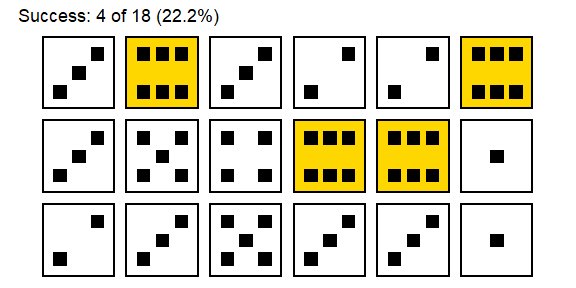#### Roll a dice once

The output of roll_dice() is a tibble. Each row represents a dice roll. Without parameters, a dice is rolled once. You can use plot_dice() to visualise the result.

set.seed(123)
roll_dice()
#> # A tibble: 1 x 5
#>   experiment round    nr result success
#>        <int> <int> <int>  <int> <lgl>
#> 1          1     1     1      3 FALSE
set.seed(123)
roll_dice() %>% plot_dice()Success is defined as result = 6 (as default), while result = 1..5 is not a success. In this case the result is 2, so it is no success.

#### Define success

If we would define result = 2 and result = 6 as success, it would be treated as success.

set.seed(123)
roll_dice(success = c(2,6))
#> # A tibble: 1 x 5
#>   experiment round    nr result success
#>        <int> <int> <int>  <int> <lgl>
#> 1          1     1     1      3 FALSE

#### Unfair dice

As default, the dice is fair. So every result (0..6) has the same probability. If you want, you can change this.

set.seed(123)
roll_dice(prob = c(0,0,0,0,0,1))
#> # A tibble: 1 x 5
#>   experiment round    nr result success
#>        <int> <int> <int>  <int> <lgl>
#> 1          1     1     1      6 TRUE

In this case we created a dice that always gets result = 6 (with 100% probability)

#### Untypically dice

As default the dice has 6 sides. If you want you can change this. Here we use a dice with 12 sides. result now can have a value between 1 and 12. But result = 6 is still the default success.

set.seed(123)
roll_dice(sides = 12)
#> # A tibble: 1 x 5
#>   experiment round    nr result success
#>        <int> <int> <int>  <int> <lgl>
#> 1          1     1     1      3 FALSE

#### Roll a dice 4 times

set.seed(123)
roll_dice(times = 4)
#> # A tibble: 4 x 5
#>   experiment round    nr result success
#>        <int> <int> <int>  <int> <lgl>
#> 1          1     1     1      3 FALSE
#> 2          1     1     2      6 TRUE
#> 3          1     1     3      3 FALSE
#> 4          1     1     4      2 FALSE
set.seed(123)
roll_dice(times = 4) %>% plot_dice()We get 1 success

#### Define rounds

set.seed(123)
roll_dice(times = 4, rounds = 2)
#> # A tibble: 8 x 5
#>   experiment round    nr result success
#>        <int> <int> <int>  <int> <lgl>
#> 1          1     1     1      3 FALSE
#> 2          1     1     2      6 TRUE
#> 3          1     1     3      3 FALSE
#> 4          1     1     4      2 FALSE
#> 5          1     2     1      2 FALSE
#> 6          1     2     2      6 TRUE
#> 7          1     2     3      3 FALSE
#> 8          1     2     4      5 FALSE
set.seed(123)
roll_dice(times = 4, rounds = 2) %>% plot_dice()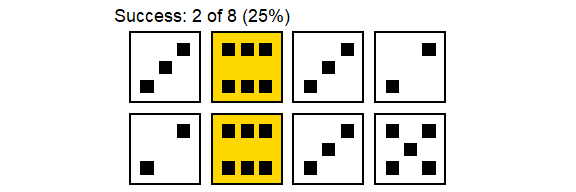Rolling the dice 4 times is repeated. In the first round we got 1 success, in the secound round 2 success.

#### Use agg

A convenient way to aggregate the result, is to use the agg parameter. Now we get one line per round.

set.seed(123)
roll_dice(times = 4, rounds = 2, agg = TRUE)
#> # A tibble: 2 x 4
#>   experiment round times success
#>        <int> <int> <int>   <int>
#> 1          1     1     4       1
#> 2          1     2     4       1

You can aggregate by hand too using dplyr.

set.seed(123)
roll_dice(times = 4, rounds = 2) %>%
group_by(experiment, round) %>%
summarise(times = n(),
success = sum(success))
#> summarise() has grouped output by 'experiment'. You can override using the
#> .groups argument.
#> # A tibble: 2 x 4
#> # Groups:   experiment 
#>   experiment round times success
#>        <int> <int> <int>   <int>
#> 1          1     1     4       1
#> 2          1     2     4       1

#### Visualise result

You can use any package/tool you like to visualise the result. In this example we use the explore-package.

set.seed(123)
roll_dice(times = 100) %>%
explore(result, title = "Rolling a dice 100x")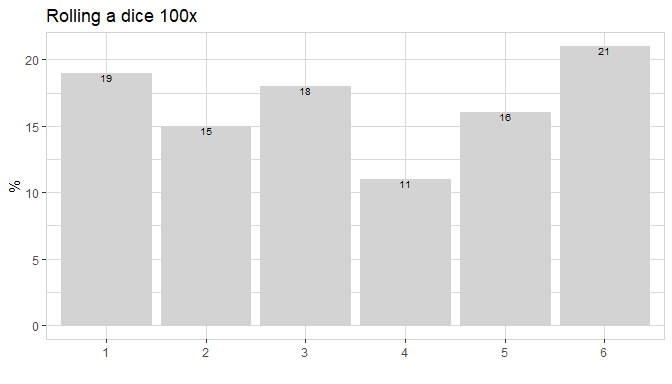In 15% of the cases we got a six. This is close to the expected value of 100/6 = 16.67%

If we increase the times parameter to 10000, the results are more balanced.

set.seed(123)
roll_dice(times = 10000) %>%
explore(result, title = "Rolling a dice 10000x")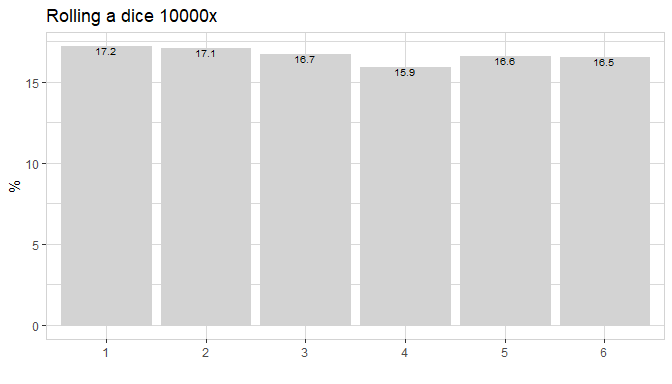#### Visualise success

If we repeat the experiment rolling a dice 100x with rounds = 100, we get the distribution with a peak at about 17 (16.67 is the expected value)

set.seed(123)
roll_dice(times = 100, rounds = 100, agg = TRUE) %>%
explore(success,
title = "Rolling 100 dice 100x",
auto_scale = FALSE)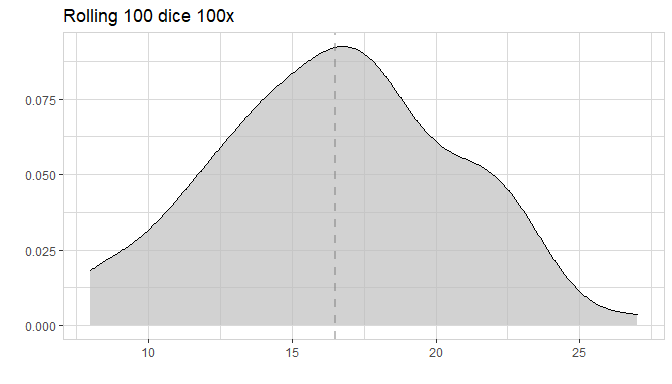If we increase rounds from 100 to 10000 we get a more symmetric shape. We see that success below 5 and success above 30 are very unlikely.

set.seed(123)
roll_dice(times = 100, rounds = 10000, agg = TRUE) %>%
explore(success,
title = "Rolling 100 dice 10000x",
auto_scale = FALSE)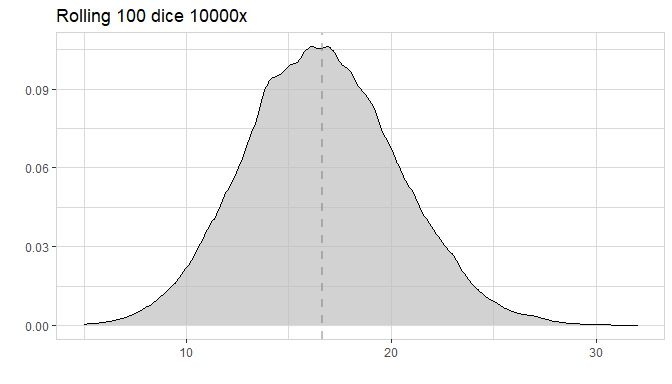This shape is already very close to the binomial distribution

binom_dice(times = 100) %>%
plot_binom(title = "Binomial distribution, rolling 100 dice")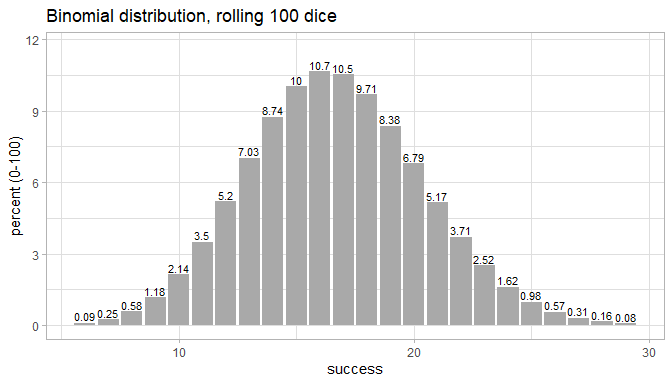set.seed(123)
roll_dice(times = 100, rounds = 10000, agg = TRUE) %>%
mutate(check = ifelse(success < 5 | success > 30, 1, 0)) %>%
count(check)
#> # A tibble: 2 x 2
#>   check     n
#>   <dbl> <int>
#> 1     0  9998
#> 2     1     2

In only 4 of 10000 (0.04%) cases success is below 5 or above 30. So the probability to get this result is very low.

We can check that with the binomial distribution too:

binom_dice(times = 100) %>%
filter(success < 5 | success > 30)
#> # A tibble: 75 x 3
#>    success            p        pct
#>      <int>        <dbl>      <dbl>
#>  1       0 0.0000000121 0.00000121
#>  2       1 0.000000241  0.0000241
#>  3       2 0.00000239   0.000239
#>  4       3 0.0000156    0.00156
#>  5       4 0.0000758    0.00758
#>  6      31 0.000172     0.0172
#>  7      32 0.0000742    0.00742
#>  8      33 0.0000306    0.00306
#>  9      34 0.0000120    0.00120
#> 10      35 0.00000454   0.000454
#> # ... with 65 more rows
binom_dice(times = 100) %>%
filter(success < 5 | success > 30) %>%
summarise(check_pct = sum(pct))
#> # A tibble: 1 x 1
#>   check_pct
#>       <dbl>
#> 1    0.0390

The probability to get this result is 0.04% (based on the binomial distribution).

#### Combine experiments

Let’s add an experiment, where you have 10 extra dice. The shape of the distribution changes.

set.seed(123)
roll_dice(times = 100, rounds = 10000, agg = TRUE) %>%
roll_dice(times = 110, rounds = 10000, agg = TRUE) %>%
explore(success,
target = experiment,
title = "Rolling a dice 100/110x",
auto_scale = FALSE)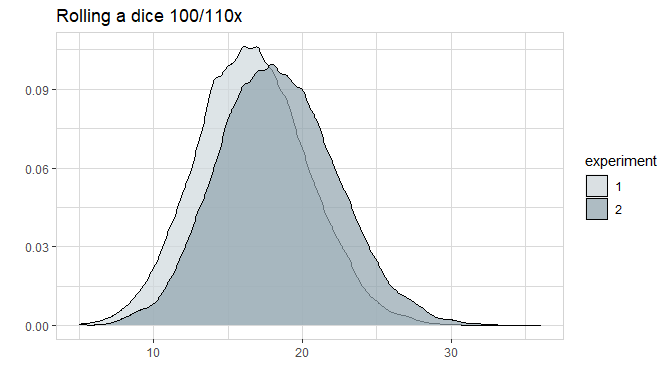You can add as many experiments as you like (as long they generate the same data structure)

Adding an experiment with times = 150 will generate a smaller but wider shape.

set.seed(123)
roll_dice(times = 100, rounds = 10000, agg = TRUE) %>%
roll_dice(times = 110, rounds = 10000, agg = TRUE) %>%
roll_dice(times = 150, rounds = 10000, agg = TRUE) %>%
explore(success,
target = experiment,
title = "Rolling a dice 100/110/150x",
auto_scale = FALSE)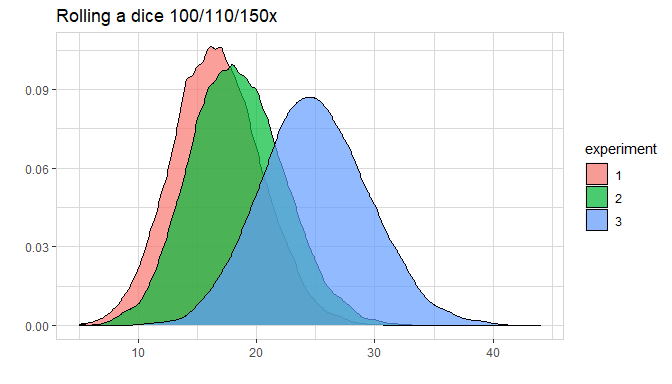#### Binomial distribution

Rolling a dice 100x, a result between 10 and 23 has a probability of over 94%

binom_dice(times = 100) %>%
plot_binom(highlight = c(10:23))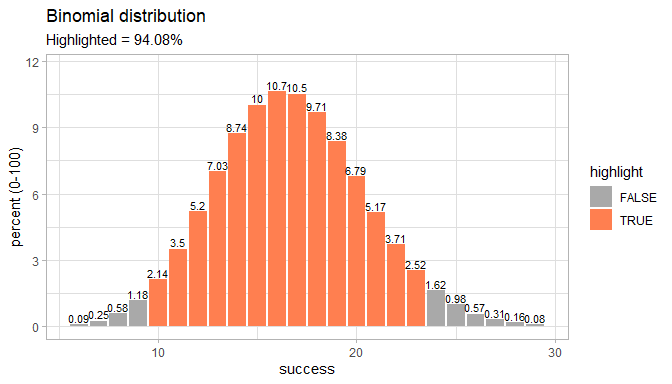### Flip a coin

Internally the package handles coins as dice with only two sides. Success is defined as result = 2 (as default), while result = 1 is not a success.

#### Flip a coin 10x

set.seed(123)
flip_coin(times = 10)
#> # A tibble: 10 x 5
#>    experiment round    nr result success
#>         <int> <int> <int>  <int> <lgl>
#>  1          1     1     1      2 TRUE
#>  2          1     1     2      2 TRUE
#>  3          1     1     3      2 TRUE
#>  4          1     1     4      2 TRUE
#>  5          1     1     5      1 FALSE
#>  6          1     1     6      1 FALSE
#>  7          1     1     7      1 FALSE
#>  8          1     1     8      2 TRUE
#>  9          1     1     9      1 FALSE
#> 10          1     1    10      1 FALSE

In this case the result are 6x 2 and 4x 1. We can use the describe() function of the explore-package to get a good overview.

set.seed(123)
flip_coin(times = 10) %>%
describe(success)
#> variable = success
#> type     = logical
#> na       = 0 of 10 (0%)
#> unique   = 2
#>    FALSE = 5 (50%)
#>     TRUE = 5 (50%)

Or just use the agg-parameter

set.seed(123)
flip_coin(times = 10, agg = TRUE)
#> # A tibble: 1 x 4
#>   experiment round times success
#>        <int> <int> <int>   <int>
#> 1          1     1    10       4

#### Define rounds

The parameter rounds can be used like in roll_dice().

set.seed(123)
flip_coin(times = 10, rounds = 4, agg = TRUE)
#> # A tibble: 4 x 4
#>   experiment round times success
#>        <int> <int> <int>   <int>
#> 1          1     1    10       4
#> 2          1     2    10       3
#> 3          1     3    10       5
#> 4          1     4    10       6

#### Visualise result

set.seed(123)
flip_coin(times = 10, rounds = 4) %>%
plot_coin()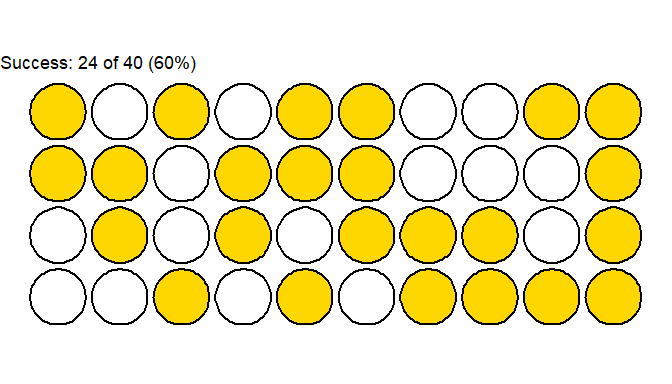#### Combine experiments

set.seed(123)
flip_coin(times = 10, agg = TRUE) %>%
flip_coin(times = 15, agg = TRUE)
#> # A tibble: 2 x 4
#>   experiment round times success
#>        <int> <int> <int>   <int>
#> 1          1     1    10       4
#> 2          2     1    15       8

#### Binomial Distribution

binom_coin(times = 10)
#> # A tibble: 11 x 3
#>    success        p     pct
#>      <int>    <dbl>   <dbl>
#>  1       0 0.000977  0.0977
#>  2       1 0.00977   0.977
#>  3       2 0.0439    4.39
#>  4       3 0.117    11.7
#>  5       4 0.205    20.5
#>  6       5 0.246    24.6
#>  7       6 0.205    20.5
#>  8       7 0.117    11.7
#>  9       8 0.0439    4.39
#> 10       9 0.00977   0.977
#> 11      10 0.000977  0.0977
binom_coin(times = 10) %>%
plot_binom(title = "Binomial distribution,\n10 coin flips")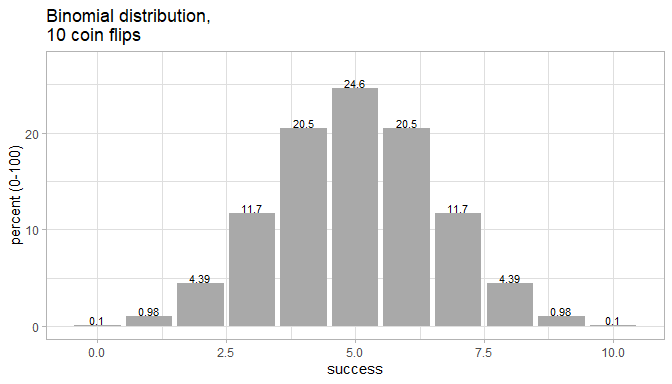### Dice design

#### Default settings

set.seed(123)
roll_dice(times = 6) %>%
plot_dice()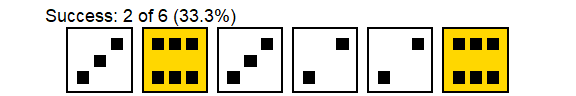#### Change color

set.seed(123)
roll_dice(times = 6) %>%
plot_dice(fill = "black", line_color = "white", point_color = "white")set.seed(123)
roll_dice(times = 6) %>%
plot_dice(fill = "lightblue", fill_success = "gold")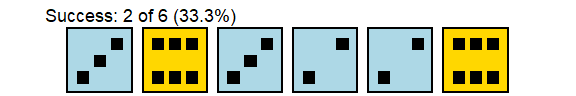set.seed(123)
roll_dice(times = 6) %>%
plot_dice(fill = "darkgrey",
fill_success = "darkblue",
line_color = "white",
point_color = "white")#### Dice type

set.seed(123)
roll_dice(times = 6) %>%
plot_dice(detailed = TRUE)set.seed(123)
roll_dice(times = 6) %>%
plot_dice(detailed = FALSE)#### Limits

plot_dice() is limited to 1 experiment with max. 10 times x 10 rounds.

set.seed(123)
roll_dice(times = 10, rounds = 10) %>%
plot_dice(detailed = FALSE, fill_success = "gold")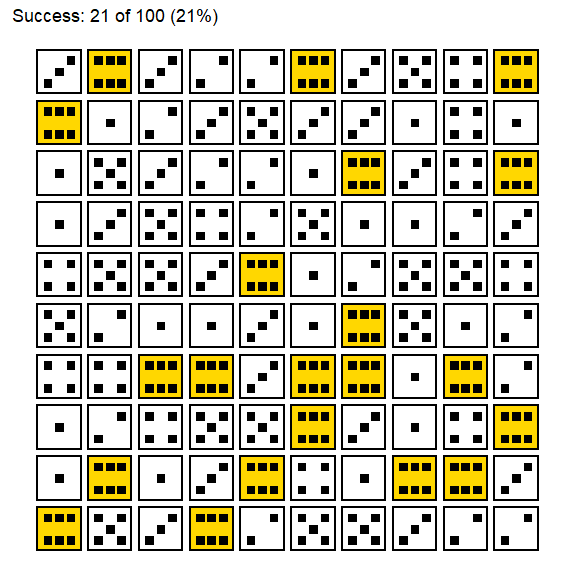#### Force

You can force a result using force_dice() and force_coin().

force_dice(1:6) %>%
plot_dice()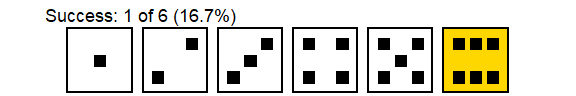force_dice(rep(6, times = 6)) %>%
plot_dice()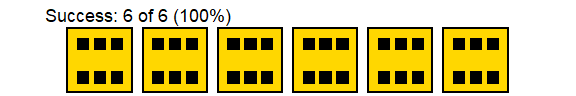We can combine two foreced dice rolling using the pipe operator and the parameter round.

force_dice(rep(5, times = 3), round = 1) %>%
force_dice(rep(6, times = 3), round = 2)
#> # A tibble: 6 x 5
#>   experiment round    nr result success
#>        <int> <int> <int>  <int> <lgl>
#> 1          1     1     1      5 FALSE
#> 2          1     1     2      5 FALSE
#> 3          1     1     3      5 FALSE
#> 4          1     2     1      6 TRUE
#> 5          1     2     2      6 TRUE
#> 6          1     2     3      6 TRUE
set.seed(123)
force_dice(rep(6, times = 3)) %>%
roll_dice(times = 3)
#> # A tibble: 6 x 5
#>   experiment round    nr result success
#>        <int> <int> <int>  <int> <lgl>
#> 1          1     1     1      6 TRUE
#> 2          1     1     2      6 TRUE
#> 3          1     1     3      6 TRUE
#> 4          2     1     1      3 FALSE
#> 5          2     1     2      6 TRUE
#> 6          2     1     3      3 FALSE

In the first experiment we get 3 times a 6 (forced), but in the second experiment none.

### Using Dice Formula

If you want to do more complex dice rolls, use roll_dice_formula()

# roll 1 dice with 6 sides
roll_dice_formula(dice_formula = "1d6", seed = 123)
#> # A tibble: 1 x 7
#>   experiment dice_formula label round    nr result success
#>        <int> <chr>        <chr> <int> <int>  <dbl> <lgl>
#> 1          1 1d6          1d6       1     1      6 TRUE
roll_dice_formula(
dice_formula = "4d6", # 4 dice with 6 sides
success = 15:24,      # success is defined as sum between 15 and 24
seed = 123            # random seed to make it reproducible
)
#> # A tibble: 1 x 7
#>   experiment dice_formula label round    nr result success
#>        <int> <chr>        <chr> <int> <int>  <dbl> <lgl>
#> 1          1 4d6          4d6       1     1     18 TRUE
# roll 4 dice with 6 sides
roll_dice_formula(
dice_formula = "4d6", # 4 dice with 6 sides
rounds = 10,          # repeat 10 times
success = 15:24,      # success is defined as sum between 15 and 24
seed = 123            # random seed to make it reproducible
)
#> # A tibble: 10 x 7
#>    experiment dice_formula label round    nr result success
#>         <int> <chr>        <chr> <int> <int>  <dbl> <lgl>
#>  1          1 4d6          4d6       1     1     13 FALSE
#>  2          1 4d6          4d6       2     1     10 FALSE
#>  3          1 4d6          4d6       3     1     14 FALSE
#>  4          1 4d6          4d6       4     1     16 TRUE
#>  5          1 4d6          4d6       5     1     12 FALSE
#>  6          1 4d6          4d6       6     1     14 FALSE
#>  7          1 4d6          4d6       7     1     15 TRUE
#>  8          1 4d6          4d6       8     1     12 FALSE
#>  9          1 4d6          4d6       9     1     15 TRUE
#> 10          1 4d6          4d6      10     1     10 FALSE
roll_dice_formula(
dice_formula = "4d6e3", # 4 dice with 6 sides, explode on a 3
rounds = 5,             # repeat 5 times
success = 15:24,        # success is defined as sum between 15 and 24
seed = 123              # random seed to make it reproducible
)
#> # A tibble: 5 x 7
#>   experiment dice_formula label round    nr result success
#>        <int> <chr>        <chr> <int> <int>  <dbl> <lgl>
#> 1          1 4d6e3        4d6e3     1     1     18 TRUE
#> 2          1 4d6e3        4d6e3     2     1     15 TRUE
#> 3          1 4d6e3        4d6e3     3     1     15 TRUE
#> 4          1 4d6e3        4d6e3     4     1     13 FALSE
#> 5          1 4d6e3        4d6e3     5     1     12 FALSE
roll_dice_formula(
dice_formula = "4d6+1d10", # 4 dice with 6 sides + 1 dice with 10 sides
rounds = 1000) %>%         # repeat 1000 times
explore_bar(result, numeric = TRUE)  # visualise result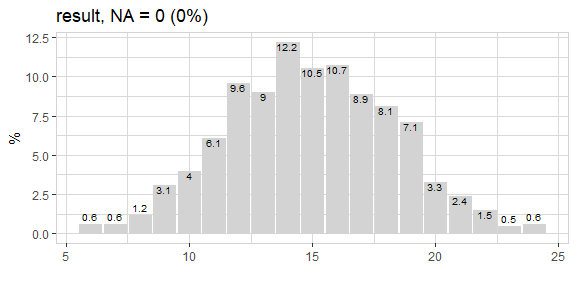Other examples for dice_formula:

• 1d6 = roll one 6-sided dice
• 1d8 = roll one 8-sided dice
• 1d12 = roll one 12-sided dice
• 2d6 = roll two 6-sided dice
• 1d6e6 = roll one 6-sided dice, explode dice on a 6
• 3d6kh2 = roll three 6-sided dice, keep highest 2 rolls
• 3d6kl2 = roll three 6-sided dice, keep lowest 2 rolls
• 4d6kh3e6 = roll four 6-sided dice, keep highest 3 rolls, but explode on a 6
• 1d20+4 = roll one 20-sided dice, and add 4
• 1d4+1d6 = roll one 4-sided dice and one 6-sided dice, and sum the results